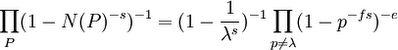## Monday, November 13, 2006

### Derivation of the Kummer Class Formula: Step One

In the next series of blogs, I will show how the Kummer Class Formula is derived from the zeta function for cyclotomic integers. In a previous blog, I showed Kummer's proof that Fermat's Last Theorem was true for regular primes. The class formula is the method for determining whether a given prime is regular or not.

The content in today's blog is taken from Harold M. Edwards
Lemma 1:

If β is an fth root of unity and n=ef, then:

(x - yβ)*...*(x - yβn) = (xf - yf)e

Proof:

(1) Xf - 1 has f distinct roots by the Fundamental Theorem of Algebra (see here)

(2) This means that:

Xf - 1 = (X - 1)(X - β)(X - β2)*...*(X - βf-1)

(3) Since βf = 1, we have:

Xf - 1 = (X - β)(X - β2)*...*(X - βf-1)(X - βf)

(4) Let X = x/y, then we have:

(x/y)f - 1 = ([x/y] - β)([x/y] - β2)*...*([x/y] - βf)

(5) If we multiply both sides by yf, we get:

xf - yf = (x - βy)(x - β2y)*...*(x - βfy)

(6) Further, if we put both sides to the power of e, we get:

(xf - yf)e = [(x - βy)(x - β2y)*...*(x - βfy)]e =

= (x - βy)(x - β2y)*...*(x - βfy)*
(x - βf+1y)(x - βf+2)*...*(x - β2fy)*
...
(x - βf(e-1)+1)(x - βf(e-1)+2)*...*(x - βefy) =

= (x - βy)*x - β2y)*...*(βny)

QED

Theorem 2:Proof:

(1) Since N(P)=pf and N(α - 1)=λ (see here for pf and here for N(α-1)=λ) and since there are e prime divisors P that divide a prime p (see here), we have:(2) For each p, we can see that:

(1 - p-fs)-e = (1f - (p-s)f)-e

(3) Let γ be a primitive root mod λ

(4) For each p, let γj ≡ p (mod λ)

(5) Let βj be an fth root of unity such that j)f = 1 but for any integer i less than f, j)f ≠ 1.

(6) Using Lemma 1 above, we have:

(1f - (p-s)f)e = (1 - βjp-s)*...*(1 - p-sβjn) = ∏ (k=1, λ-1) (1 - βjkp-s) =

=∏ (k=0, λ-2) (1 - β
jkp-s)

(7) Using step #6, we have:

(1 - λ-s)-1 ∏ (p ≠ λ) (1 - p-fs)-e = (1 - λ-s)-1 ∏ (p ≠ λ) ∏ (k=0, λ-2) (1 - p-sβjk)-1

(8) From this step, the conclusion follows:QED

References